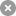1. Home
2. TOOLBOX
3. Energy Calculator

# Energy CalculatorUsing this energy calculator you may determine how much energy an JIXIANG solar collector will produce each day/month/year. The way you utilize this energy is up to you. You can heat water for showering and washing clothes, or central heat a building. In fact one integrated system can complete both these functions.

You can also use these values to help you calculate how much energy you can save by using an JIXIANG solar collector.

In order to calculate energy output you must input the following variables:
Insolation Level - Before you calculate your energy output, you must know your solar insolation level. This are available from the insolation page. Take note of your max and min levels throughout the year as well as the annual average value. When assessing potential energy savings, input annual average insolation, and take note of the "per year" energy output value.
Energy must be input in the unit kWh/m2/day. 1 kWh/m2/day = 317.1 Btu/ft2/day

Collector Size - You must enter the collector size in absorber surface area.
The absorber surface area of the various tubes sizes are as follows:
- 58/1800 = 0.08m2 per tube. Therefore an JIXIANG AP 20 tube = 1.6m2 absorber area
- 58/1500 = 0.067m2 per tube
Energy Cost - Enter cost per kWh in your local currency
(may need to convert from m3 or Therms)
1 therm = 29.3kWh = 100,000Btu = 105.5MJ
Natural Gas is 39MJ/m3 = 10.83 kWh/m3
LPG Propane (liquid) = 25.3MJ/L = 7kWh/L
LPG Propane (gas) = 93.3MJ/m3 = 25.9kWh/m3
 Insolation Level = kWh/m2/day Absorber Area = m2 Electricity Cost = \$/£/€ per kWh (click twice) Energy Output Per Day = kWh Per Month = kWh Per Year = kWh = Btu = Btu = Btu = \$/£/€ = \$/£/€ = \$/£/€
- Collector peak efficiency is only achieved when ambient temperature and water temperatures are the same. During normal use, this is only likely to happen for a short period of time each day, and usually only when ambient temperatures are high (summer). Therefore during normal use, the solar collector can not always perform at such a high level of efficiency. This is true for all evacuated tube and flat plate collectors, not only JIXIANG collectors. In order to provide more realistic figures, the above calculations are based on "normal" operating conditions under which the difference between ambient temp and manifold water temp is around 30-40oC.

- When making comparisons with other products please take the above point into consideration. Do not simply use the peak efficiency values for energy output, as this will provide inflated figures. IAM values also play an important role in determining total energy output from a solar collector. Please click here to learn more about how to interpret IAM figures.

- Monthly and annual values are calculated using 28 days and 336 days respectively to account for days of very low solar radiation.

- Energy output values are approximations. Actual energy output and overall system efficiency will depend upon installation location, climate, insulation, system configuration and many other factors. On rainy or heavily overcast days energy output will be greatly reduced.

- Energy is produced in the form of heat. In transporting and converting this energy, such as for air conditioning or central heating, some energy (heat) will be lost, as no system or insulation is 100% efficient.
Top
Scan the QR code Close
Scan the QR code Close

### Send Message

*****
Choose language